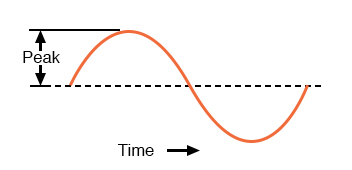## How do you measure the intensity or magnitude of an AC quantity?

Measure its peak height on a waveform graph.

# Measurements of AC Magnitude

## Chapter 1 - Basic AC Theory

So far we know that AC voltage alternates in polarity and AC current alternates in direction. We also know that AC can alternate in a variety of different ways, and by tracing the alternation over time we can plot it as a “waveform.”

We can measure the rate of alternation by measuring the time it takes for a wave to evolve before it repeats itself (the “period”), and express this as cycles per unit time, or “frequency.” In music, frequency is the same as pitch, which is the essential property distinguishing one note from another.

However, we encounter a measurement problem if we try to express how large or small an AC quantity is. With DC, where quantities of voltage and current are generally stable, we have little trouble expressing how much voltage or current we have in any part of a circuit.

But how do you grant a single measurement of magnitude to something that is constantly changing?

### Ways of Expressing the Magnitude of an AC Waveform

One way to express the intensity, or magnitude (also called the amplitude), of an AC quantity is to measure its peak height on a waveform graph. This is known as the peak or crest value of an AC waveform: Figure below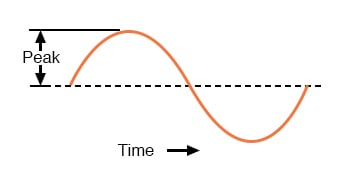Peak voltage of a waveform.

Another way is to measure the total height between opposite peaks. This is known as the peak-to-peak (P-P) value of an AC waveform: Figure below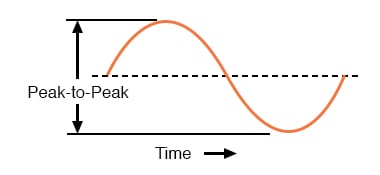Peak-to-peak voltage of a waveform.

Unfortunately, either one of these expressions of waveform amplitude can be misleading when comparing two different types of waves. For example, a square wave peaking at 10 volts is obviously a greater amount of voltage for a greater amount of time than a triangle wave peaking at 10 volts.

The effects of these two AC voltages powering a load would be quite different: Figure below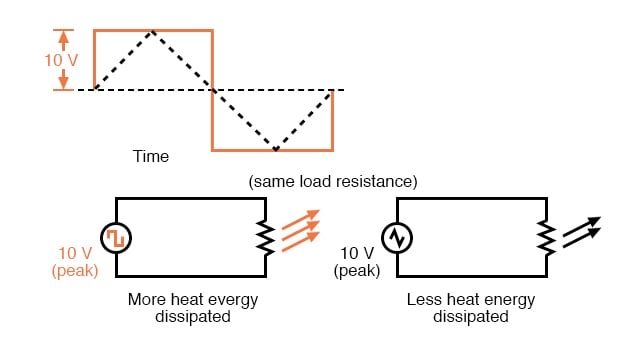A square wave produces a greater heating effect than the same peak voltage triangle wave.

One way of expressing the amplitude of different wave shapes in a more equivalent fashion is to mathematically average the values of all the points on a waveform’s graph to a single, aggregate number. This amplitude measurement is known simply as the average value of the waveform.

If we average all the points on the waveform algebraically (that is, to consider their sign, either positive or negative), the average value for most waveforms is technically zero, because all the positive points cancel out all the negative points over a full cycle: Figure below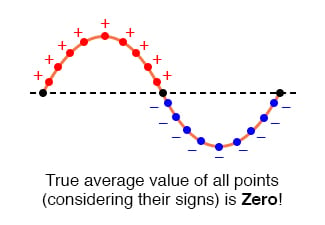The average value of a sine wave is zero.

This, of course, will be true for any waveform having equal-area portions above and below the “zero” line of a plot. However, as a practical measure of a waveform’s aggregate value, “average” is usually defined as the mathematical mean of all the points’ absolute values over a cycle.

In other words, we calculate the practical average value of the waveform by considering all points on the wave as positive quantities, as if the waveform looked like this: Figure below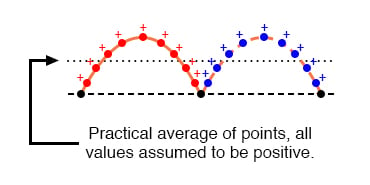Waveform seen by AC “average responding” meter.

Polarity-insensitive mechanical meter movements (meters designed to respond equally to the positive and negative half-cycles of an alternating voltage or current) register in proportion to the waveform’s (practical) average value, because the inertia of the pointer against the tension of the spring naturally averages the force produced by the varying voltage/current values over time.

Conversely, polarity-sensitive meter movements vibrate uselessly if exposed to AC voltage or current, their needles oscillating rapidly about the zero mark, indicating the true (algebraic) average value of zero for a symmetrical waveform. When the “average” value of a waveform is referenced in this text, it will be assumed that the “practical” definition of average is intended unless otherwise specified.

Another method of deriving an aggregate value for waveform amplitude is based on the waveform’s ability to do useful work when applied to a load resistance. Unfortunately, an AC measurement based on work performed by a waveform is not the same as that waveform’s “average” value, because the power dissipated by a given load (work performed per unit time) is not directly proportional to the magnitude of either the voltage or current impressed upon it.

Rather, power is proportional to the square of the voltage or current applied to a resistance (P = E2/R, and P = I2R). Although the mathematics of such an amplitude measurement might not be straightforward, the utility of it is.

Consider a bandsaw and a jigsaw, two pieces of modern woodworking equipment. Both types of saws cut with a thin, toothed, motor-powered metal blade to cut wood. But while the bandsaw uses a continuous motion of the blade to cut, the jigsaw uses a back-and-forth motion.

The comparison of alternating current (AC) to direct current (DC) may be likened to the comparison of these two saw types: Figure below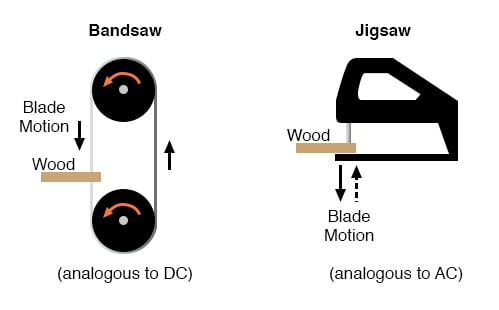Bandsaw-jigsaw analogy of DC vs AC.

The problem of trying to describe the changing quantities of AC voltage or current in a single, aggregate measurement is also present in this saw analogy: how might we express the speed of a jigsaw blade? A bandsaw blade moves with a constant speed, similar to the way DC voltage pushes or DC current moves with a constant magnitude. A jigsaw blade, on the other hand, moves back and forth, its blade speed constantly changing. What is more, the back-and-forth motion of any two jigsaws may not be of the same type, depending on the mechanical design of the saws.

One jigsaw might move its blade with a sine-wave motion, while another with a triangle-wave motion. To rate a jigsaw based on its peak blade speed would be quite misleading when comparing one jigsaw to another (or a jigsaw with a bandsaw!). Despite the fact that these different saws move their blades in different manners, they are equal in one respect: they all cut wood, and a quantitative comparison of this common function can serve as a common basis for which to rate blade speed.

Picture a jigsaw and bandsaw side-by-side, equipped with identical blades (same tooth pitch, angle, etc.), equally capable of cutting the same thickness of the same type of wood at the same rate. We might say that the two saws were equivalent or equal in their cutting capacity. Might this comparison be used to assign a “bandsaw equivalent” blade speed to the jigsaw’s back-and-forth blade motion; to relate the wood-cutting effectiveness of one to the other?

This is the general idea used to assign a “DC equivalent” measurement to any AC voltage or current: whatever magnitude of DC voltage or current would produce the same amount of heat energy dissipation through an equal resistance: Figure below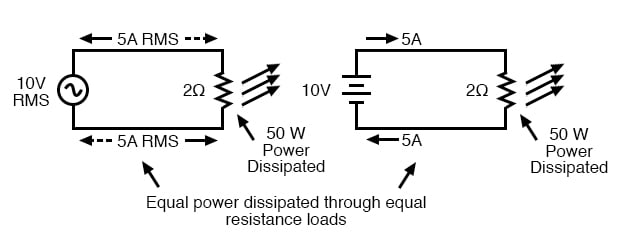An RMS voltage produces the same heating effect as the same DC voltage

### How is Root Mean Square (RMS) Relevant to AC?

In the two circuits above, we have the same amount of load resistance (2 Ω) dissipating the same amount of power in the form of heat (50 watts), one powered by AC and the other by DC. Because the AC voltage source pictured above is equivalent (in terms of power delivered to a load) to a 10 volt DC battery, we would call this a “10 volt” AC source.

More specifically, we would denote its voltage value as being 10 volts RMS. The qualifier “RMS” stands for Root Mean Square, the algorithm used to obtain the DC equivalent value from points on a graph (essentially, the procedure consists of squaring all the positive and negative points on a waveform graph, averaging those squared values, then taking the square root of that average to obtain the final answer).

Sometimes the alternative terms equivalent or DC equivalent are used instead of “RMS,” but the quantity and principle are both the same.

RMS amplitude measurement is the best way to relate AC quantities to DC quantities, or other AC quantities of differing waveform shapes, when dealing with measurements of electric power.

For other considerations, peak or peak-to-peak measurements may be the best to employ. For instance, when determining the proper size of wire (ampacity) to conduct electric power from a source to a load, RMS current measurement is the best to use, because the principal concern with current is overheating of the wire, which is a function of power dissipation caused by current through the resistance of the wire.

However, when rating insulators for service in high-voltage AC applications, peak voltage measurements are the most appropriate, because the principal concern here is insulator “flashover” caused by brief spikes of voltage, irrespective of time.

### Instruments Used to Measure the Amplitude of a Waveform

Peak and peak-to-peak measurements are best performed with an oscilloscope, which can capture the crests of the waveform with a high degree of accuracy due to the fast action of the cathode-ray-tube in response to changes in voltage. For RMS measurements, analog meter movements (D’Arsonval, Weston, iron vane, electrodynamometer) will work so long as they have been calibrated in RMS figures.

Because the mechanical inertia and dampening effects of an electromechanical meter movement makes the deflection of the needle naturally proportional to the average value of the AC, not the true RMS value, analog meters must be specifically calibrated (or mis-calibrated, depending on how you look at it) to indicate voltage or current in RMS units.

The accuracy of this calibration depends on an assumed waveshape, usually a sine wave.

Electronic meters specifically designed for RMS measurement are best for the task. Some instrument manufacturers have designed ingenious methods for determining the RMS value of any waveform. One such manufacturer produces “True-RMS” meters with a tiny resistive heating element powered by a voltage proportional to that being measured.

The heating effect of that resistance element is measured thermally to give a true RMS value with no mathematical calculations whatsoever, just the laws of physics in action in fulfillment of the definition of RMS. The accuracy of this type of RMS measurement is independent of waveshape.

### Relationship of Peak, Peak-to-Peak, Average, and RMS

For “pure” waveforms, simple conversion coefficients exist for equating Peak, Peak-to-Peak, Average (practical, not algebraic), and RMS measurements to one another: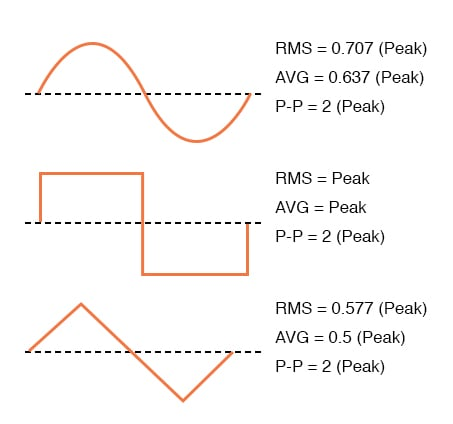Conversion factors for common waveforms.

In addition to RMS, average, peak (crest), and peak-to-peak measures of an AC waveform, there are ratios expressing the proportionality between some of these fundamental measurements. The crest factor of an AC waveform, for instance, is the ratio of its peak (crest) value divided by its RMS value.

The form factor of an AC waveform is the ratio of its RMS value divided by its average value. Square-shaped waveforms always have crest and form factors equal to 1, since the peak is the same as the RMS and average values. Sinusoidal waveforms have an RMS value of 0.707 (the reciprocal of the square root of 2) and a form factor of 1.11 (0.707/0.636).

Triangle- and sawtooth-shaped waveforms have RMS values of 0.577 (the reciprocal of square root of 3) and form factors of 1.15 (0.577/0.5).

Bear in mind that the conversion constants shown here for peak, RMS, and average amplitudes of sine waves, square waves, and triangle waves hold true only for pure forms of these waveshapes. The RMS and average values of distorted waveshapes are not related by the same ratios: Figure below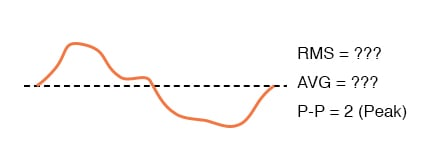Arbitrary waveforms have no simple conversions.

This is a very important concept to understand when using an analog D’Arsonval meter movement to measure AC voltage or current. An analog D’Arsonval movement, calibrated to indicate sine-wave RMS amplitude, will only be accurate when measuring pure sine waves.

If the waveform of the voltage or current being measured is anything but a pure sine wave, the indication given by the meter will not be the true RMS value of the waveform, because the degree of needle deflection in an analog D’Arsonval meter movement is proportional to the average value of the waveform, not the RMS.

RMS meter calibration is obtained by “skewing” the span of the meter so that it displays a small multiple of the average value, which will be equal to be the RMS value for a particular waveshape and a particular waveshape only.

Since the sine-wave shape is most common in electrical measurements, it is the waveshape assumed for analog meter calibration, and the small multiple used in the calibration of the meter is 1.1107 (the form factor: 0.707/0.636: the ratio of RMS divided by average for a sinusoidal waveform).

Any waveshape other than a pure sine wave will have a different ratio of RMS and average values, and thus a meter calibrated for sine-wave voltage or current will not indicate true RMS when reading a non-sinusoidal wave. Bear in mind that this limitation applies only to simple, analog AC meters not employing “True-RMS” technology.

REVIEW:

• The amplitude of an AC waveform is its height as depicted on a graph over time. An amplitude measurement can take the form of peak, peak-to-peak, average, or RMS quantity.
• Peak amplitude is the height of an AC waveform as measured from the zero mark to the highest positive or lowest negative point on a graph. Also known as the crest amplitude of a wave.
• Peak-to-peak amplitude is the total height of an AC waveform as measured from maximum positive to maximum negative peaks on a graph. Often abbreviated as “P-P”.
• Average amplitude is the mathematical “mean” of all a waveform’s points over the period of one cycle. Technically, the average amplitude of any waveform with equal-area portions above and below the “zero” line on a graph is zero. However, as a practical measure of amplitude, a waveform’s average value is often calculated as the mathematical mean of all the points’ absolute values (taking all the negative values and considering them as positive). For a sine wave, the average value so calculated is approximately 0.637 of its peak value.
• “RMS” stands for Root Mean Square, and is a way of expressing an AC quantity of voltage or current in terms functionally equivalent to DC. For example, 10 volts AC RMS is the amount of voltage that would produce the same amount of heat dissipation across a resistor of given value as a 10 volt DC power supply. Also known as the “equivalent” or “DC equivalent” value of an AC voltage or current. For a sine wave, the RMS value is approximately 0.707 of its peak value.
• The crest factor of an AC waveform is the ratio of its peak (crest) to its RMS value.
• The form factor of an AC waveform is the ratio of its RMS value to its average value.
• Analog, electromechanical meter movements respond proportionally to the average value of an AC voltage or current. When RMS indication is desired, the meter’s calibration must be “skewed” accordingly. This means that the accuracy of an electromechanical meter’s RMS indication is dependent on the purity of the waveform: whether it is the exact same waveshape as the waveform used in calibrating.

RELATED WORKSHEETS:

• Share
Published under the terms and conditions of the Design Science License
1 Comment